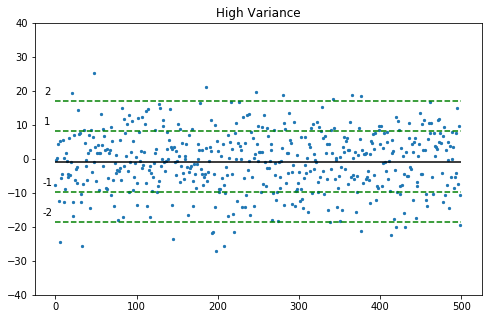Standard deviation is the amount of variance you have in your data. It is measured in the same units as your data points (dollars, temperature, minutes, etc.). To find standard deviation in pandas, you simply call .std() on your Series or DataFrame

```pandas.DataFrame.std()
pandas.Series.std()```

I do this most often when I’m working with anomaly detection. I’m trying to find the outliers of a specific dataset. For example: If I’m looking at a time series of temperature readings per day, which days were ‘out of the ordinarily hot’? Looking at standard deviation would help me with this.

Pseudo Code: With your Series or DataFrame, find how much variance, or how spread out, your data points are.

## Pandas Standard Deviation

Standard deviation describes how much variance, or how spread out your data is. In the picture below, the chart on the left does not have a wide spread in the Y axis. Meaning the data points are close together. This is called low standard deviation.

The chart on the right has high spread of data in the Y Axis. The data points are spread out. This would mean there is a high standard deviation.

### Pandas STD Parameters

The standard deviation function is pretty standard, but you may want to play with a view items.

• axis = Do you want to compute the standard deviation across rows? or or columns? Index (rows) = 0, columns = 1
• skipna = By default, Pandas will skip the NAs in your dataset. If you set skipna=False, make sure you understand how your NAs are impacting your results.
• level = For when you have a multi index. 95% of the time this won’t matter because you’ll be on a single index. If not, then set your level to the level you want to compute the STD for.
• Others: For the other lesser-used parameters, see the official documentation.

Now the fun part, let’s take a look at a code sample

In :
```import pandas as pd
import matplotlib.pyplot as plt
import numpy as np # do help with random numbers
np.random.seed(seed=42)
```

### Pandas Standard Deviation¶

Standard Deviation is the amount of 'spread' you have in your data. More variance, more spread, more standard deviation.

I like to see this explained visually, so let's create charts

Let's first create a DataFrame with two columns. One with low variance, one with high variance.

I'm going to create these via numpy random number generator. The important part is to look at the charts.

Examples to run through

1. Calculating standard deviation on a Series
2. Calculating standard deviation on a DataFrame
In :
```data_points = 500
df = pd.DataFrame({'low_var': np.random.normal(loc=0, scale=2, size=data_points),
'high_var': np.random.normal(loc=0, scale=9, size=data_points)})
```
Out:
low_varhigh_var
02.361282-7.771442
1-1.254627-0.280831
20.0904450.162152
30.1023964.253673
4-1.003568-12.301725

Then let's visualize our data. I'm going to plot the points on a scatter plot, and also plot the mean as a horizontal line

In :
```plt.ylim(-40,40) # Setting y limits so the axis are consistent
plt.title("Low Variance") # Setting the title
plt.scatter(x=df.index, y=df['low_var'], s=5); # Plotting the scatter
plt.hlines(y=df['low_var'].mean(), xmin=0, xmax=data_points) # Mean line
plt.show(); # Telling matplotlib to show the chart

plt.title("High Variance")
plt.ylim(-40,40)
plt.scatter(x=df.index, y=df['high_var'], s=5);
plt.hlines(y=df['high_var'].mean(), xmin=0, xmax=data_points);
```### 1. Calculating Standard Deviation on a Series¶

Let's calc std on a pandas series. Do to this, simply call .std() on your Series.

In :
```df['low_var'].std()
```
Out:
`2.0335824820605577`
In :
```df['high_var'].std()
```
Out:
`8.924455248568384`

### 2. Calculating Standard Deviation on a DataFrame¶

You can also apply this function directly to a DataFrame so it will do the std of all the columns

In :
```df.std()
```
Out:
```low_var     2.033582
high_var    8.924455
dtype: float64```

### 3. Extra: Plotting 1 & 2 standard deviations from the mean¶

Standard Deviation is used in outlier detection. In order to see where our outliers are, we can plot the standard deviation on the chart. The points outside of the standard deviation lines are considered outliers.

In :
```plt.figure(figsize=(8,5))
plt.title("High Variance") # Title
plt.ylim(-40,40) # Setting y limits
plt.scatter(x=df.index, y=df['high_var'], s=5); # Plotting scatter
plt.hlines(y=df['high_var'].mean(), xmin=0, xmax=data_points) # Mean

for std_int in [-2, -1, 1, 2]: # Going through different stds from the mean
standard_deviation = df['high_var'].mean() + df['high_var'].std()*std_int

plt.hlines(y=standard_deviation,
xmin=0,
xmax=data_points,
linestyles='dashed',
colors='green'); # 1 std above

# Giving labels to the lines we just drew
plt.text(y=standard_deviation + 2, x=-10, s=std_int, ha='center')
```In [ ]:
```
```